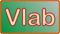onlineoverland:   Overland flow using the diffusion wave method --- One book --- [Kinematic hydraulic diffusivity 211002]References Ponce, V. M. 1986. Diffusion wave modeling of catchment dynamics. Journal of Hydraulic Engineering, 112(8), August, 716-727. [HTML version 2015]. Ponce, V. M. 1989. Engineering Hydrology: Principles and Practices. Prentice Hall, Englewood Cliffs, New Jersey, 322-325. Aguilar, R. D. 2014. Diffusion wave modeling of catchment dynamics using online calculation.

 INPUT DATA: [Rationale] [Description] [Sample Input]

Select:

 SI units (metric) U.S. Customary units

(SI (metric) / U.S. Customary)

Rainfall depth P  (cm / in):

cm
[ P > 0 ]

Runoff curve number CN :

[ CN > 20 ]

Total rainfall duration tr  (hr):

[ tr > 0 ]

Number of points in cumulative rainfall distribution Np :

[ Integer Np must be in the range 2 ≤ Np ≤ 101 ]

Dimensionless cumulative time for rainfall distribution T* :

[Np values, separated by commas; first value must be 0.; last value must be 1.]

Dimensionless cumulative depth for rainfall distribution D* :

[Np values, separated by commas; first value must be 0.; last value must be 1.]

Total simulation time Tt  (hr):

[ Tt > 0 ]

Number of time intervals NΔt :

[ Integer NΔt > 0 ]

Number of time intervals for printing Npr :

[ Integer Npr > 0 ]

Fraction used to estimate reference discharge Fr :

[ 0 ≤ Fr ≤ 1; leave blank to specify default value of Fr = 0.5 ]

Overland flow area A  (ha / ac):

ha
[ 0 < A ≤ 2500 in SI Units; 0 < A ≤ 1000 in U.S. Units ]

Fraction of area in left plane Flp :

[ 0 < Flp ≤ 1; enter 1 to specify only one (left) plane ]

Slope of left plane Slp :

[ 0.000001 ≤ Slp ≤ 0.1 ]

Manning coefficient for left plane nlp :

[ 0.008 ≤ nlp ≤ 1 ]

Rating exponent for left plane βlp :

[ 1.5 ≤ βlp ≤ 3; enter -1 to specify default value of βlp = 2 ]

Slope of right plane Srp :

[ 0.000001 ≤ Srp ≤ 0.1; leave blank to exactly mirror left plane for Flp = 0.5 only ]

Manning coefficient for right plane nrp :

[ 0.008 ≤ nrp ≤ 1; leave blank to exactly mirror left plane for Flp = 0.5 only ]

Rating exponent for right plane βrp :

[ 1.5 ≤ βrp ≤ 3; enter -1 to specify default value of βlp = 2;
leave blank to exactly mirror left plane for Flp = 0.5 only ]

Length of channel Lch  (m / ft):

m
[ Lch > 0 ]

Slope of channel Sch :

[ 0.000001 ≤ Sch ≤ 0.1 ]

Manning coefficient of channel nch :

[ 0.008 ≤ nch ≤ 0.250 ]

Bottom width of channel Bch  (m / ft):

m
[ Bch ≥ 0 ]

Design depth of channel ych  (m / ft):

m
[ ych > 0 ]

Side slope of channel z [z H : 1 V]:

[ 0 ≤ z ≤ 10 ]

OUTPUT:

 Time step Time(sec) Time(min) Time(hr) Outflow(m3/s) Cumulative Volume(m3) 0 0 0 0 0 0

Your request was processed at  08:36:34 pm on January 28th, 2022   [ 220128  20:36:34 ].

Thank you for running   onlineoverland.php   Please call again.